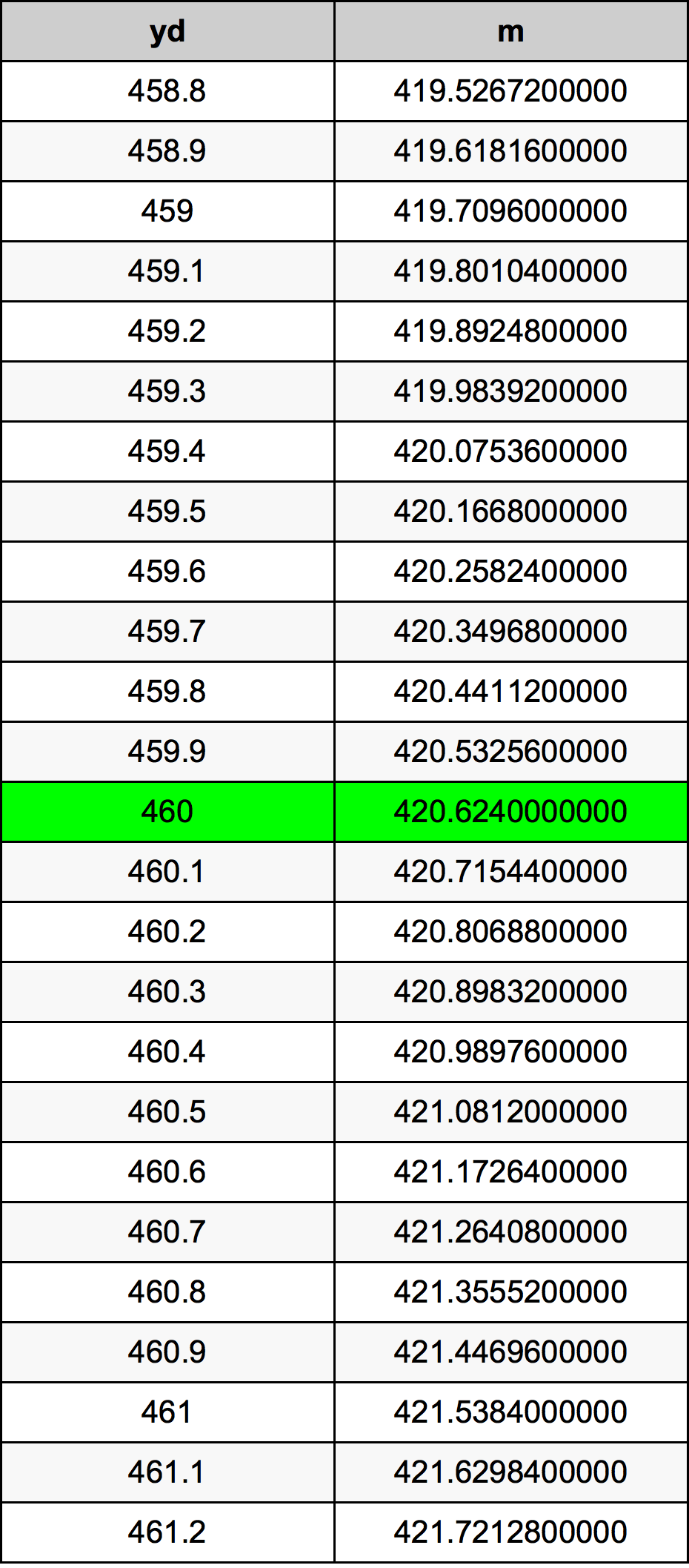Yards To Meters

# 460 yd to m460 Yards to Meters

yd
=
m

## How to convert 460 yards to meters?

 460 yd * 0.9144 m = 420.624 m 1 yd
A common question is How many yard in 460 meter? And the answer is 503.062117235 yd in 460 m. Likewise the question how many meter in 460 yard has the answer of 420.624 m in 460 yd.

## How much are 460 yards in meters?

460 yards equal 420.624 meters (460yd = 420.624m). Converting 460 yd to m is easy. Simply use our calculator above, or apply the formula to change the length 460 yd to m.

## Convert 460 yd to common lengths

UnitUnit of length
Nanometer4.20624e+11 nm
Micrometer420624000.0 µm
Millimeter420624.0 mm
Centimeter42062.4 cm
Inch16560.0 in
Foot1380.0 ft
Yard460.0 yd
Meter420.624 m
Kilometer0.420624 km
Mile0.2613636364 mi
Nautical mile0.2271187905 nmi

## What is 460 yards in m?

To convert 460 yd to m multiply the length in yards by 0.9144. The 460 yd in m formula is [m] = 460 * 0.9144. Thus, for 460 yards in meter we get 420.624 m.

## 460 Yard Conversion Table## Alternative spelling

460 yd to m, 460 yd in m, 460 yd to Meters, 460 yd in Meters, 460 yd to Meter, 460 yd in Meter, 460 Yard to m, 460 Yard in m, 460 Yard to Meter, 460 Yard in Meter, 460 Yard to Meters, 460 Yard in Meters, 460 Yards to m, 460 Yards in m